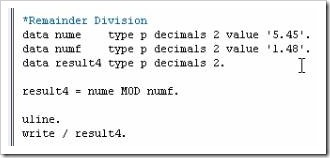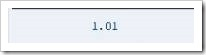# 6 Learning Tips For Coding SAP ABAP Calculations

Now that the ability to create variables has been established, these can be used for calculations within a program. This article will begin by looking at some of the simple arithmetical calculations within ABAP.

Our program will be tidied up by removing the two constants which were just created. If a program needs to add two numbers together and each number is stored as its own unique variable, the product of the two numbers can be stored in a brand new variable titled “result”.

Create a new DATA statement, name this “result” and use the LIKE statement to give it the same properties as packed_decimal01, terminating the line with a period.

To add two numbers together, on a new line, type “result = integer01 + packed_decimal01.” On a new line enter, “WRITE result.” Activate and test the program, and the result will appear in the output screen: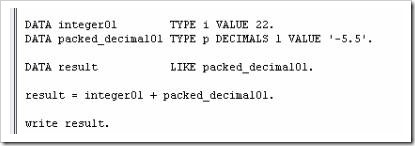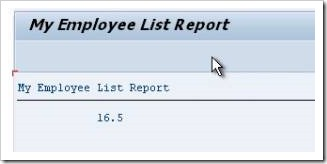Things to remember: For any arithmetical operation, the calculation itself must appear to the right of the =, and the variable to hold the result to the left. This ensures that only the result variable will be updated in the execution. If the variable titled “result” had been assigned a value prior to the calculation, this would be overwritten with the new value. Spaces must always be inserted on either side of the = and + signs. This applies to all arithmetical operators, including parentheses ( ), which will start to be used as the calculations become more complicated. Note that one space is the minimum, and multiple spaces can be used, which may help in lining code up to make it more readable, and indeed where calculations may be defined over many lines of code.

It is not just the products of variables which can be calculated in calculations, but also individual literal values, or a mixture of the two, as shown here: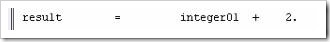## Arithmetic – Subtraction

To subtract numbers, the same method is used, replacing the + with a . Copy and paste the previous calculation and make this change. Also, to make this simpler to understand, change the value of packed_decimal01 from -5.5 to 5.5. One can see by doing this the way that changing the initial variable will alter the calculation.

Execute the code: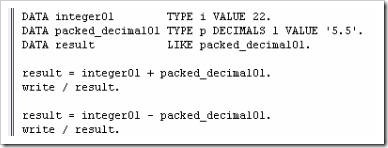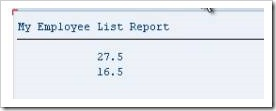## Arithmetic – Division

To divide numbers, the same method is followed, but the arithmetical operator this time will be a /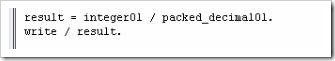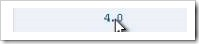## Arithmetic – Multiplication

To multiply, the operator is a *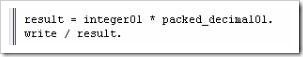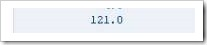Additionally to these methods, the statements ADD, SUBTRACT, DIVIDE and MULTIPLY can be used. The syntax for these is slightly different. Beneath the first calculation (where integer01 and packed_decimal01 where added), write a new line of code
ADD 8 to result.” (Ignore the comment line in the image):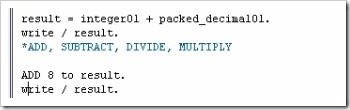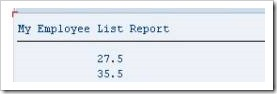While this is a legitimate method for calculations, it must be added that this is very rarely used, as the initial method is much simpler.

## Conversion Rules

In this program, different data types have been used when declaring variables. It is the responsibility of the programmer to ensure the data types used are compatible with one another when used for calculations or moving data to and from objects. One should not attempt calculations with variables and numbers which do not match.

For example, a variable defined as an integer cannot be multiplied by a character, as these two data types are incompatible. This would cause the system to generate syntax and runtime errors when the program is executed. While SAP has built in automatic data type conversions for many of the standard data types within ABAP, there are scenarios where the inbuilt conversion rules are not appropriate. It is important to become familiar with the inbuilt conversion rules and know when to manipulate the data prior to using them in calculations. Here, some examples of conversion rules will be given, so that they can be used throughout programs created.

Conversion rules are pre-defined logic that determine how the contents of the source field can be entered into a target field. If one attempts to insert an integer field containing the value of 1 to a character string, the built-in conversion rules will determine exactly how this should be done without any syntax or runtime errors.

For example, create a DATA statement with the name “num1” of TYPE p (packed decimal) with DECIMALS 2 and a VALUE of ‘3.33’. Then create another variable with the name “result1” of type i (integer). Attempt the calculation “result1 = num1”. The conversion rule here would round the number to the closest integer, in this case 3.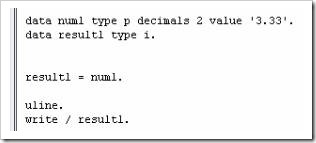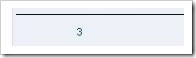As you work with different data types, these kinds of conversion rules will often be applied automatically, and it is up to you, the programmer, to understand these conversion rules and the data types used within the program to ensure no runtime errors occur.

## Division Variations

Now, a slight step back will be taken to discuss the division operator further. In ABAP, there are three ways in which numbers can be divided:

· The standard result with decimal places

· The remainder result

· The integer result.

### The standard form of division.

Create 2 variables, “numa” and “numb”, with values of 5.45 and 1.48 respectively, then create the variable “result2” (also with 2 decimal places). Then insert the calculation “result2 = numa / numb.” followed by a WRITE statement for result2. Execute the program.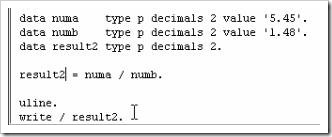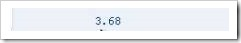### The integer form of division.

Copy the initial calculation; change the initial variables to “numc” and “numd” and the resulting variable to “result3”. For integer division, rather than using the standard /, use the operator DIV. This will give the result of the calculation’s integer value, without the decimal places.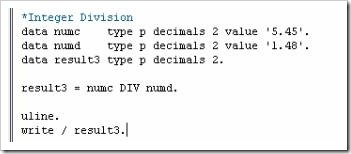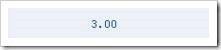### The remainder form of division.

Follow the steps from the integer form, this time with “nume”, “numf” and “result4”. For this type of division, the arithmetical operator should be MOD. This, when executed, will show the remainder value.